## Tuesday, September 13, 2011

### Sharp EL-W516X Review

Hi everyone. Today I am giving a short review of the Sharp EL-W516X solar calculator. I bought this calculator at Target for \$17.99.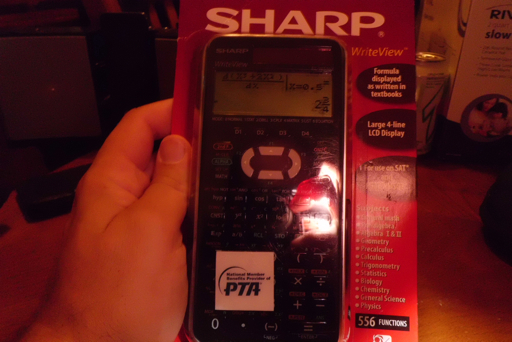The main features of the EL-W516X include: WriteView mode, statistical operations, matrix operations, base calculations, complex operations, and a drill mode. The drill mode tests your mathematical ability on arithmetic problems. While this mode has been panned, I find the drill mode enjoyable, and I challenge myself to see how fast I can correctly answer a set of questions.

Generally, this calculator is a remake of the Sharp EL-W516 calculator. A picture of both models are shown below, with the newer EL-W516X on the left, and the older EL-W516 on the right.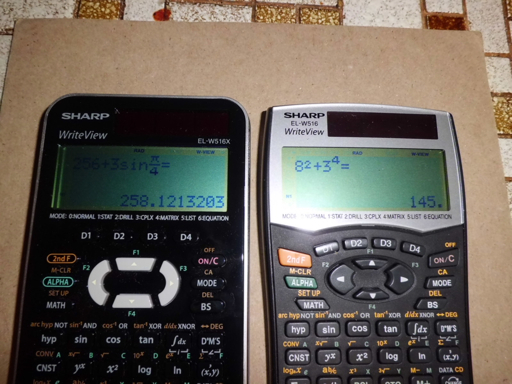The set of operations of the EL-W516X is the same as the EL-W516. The normal mode allows you enter calculations in a linear format or a textbook format (WriteView ™). The textbook format reurns exact answers (fractions, fractions of π, square roots) whenever possible. Decimal equivalents can be accessed by pressing the CHANGE key (sometimes twice).

Some of them include:

Catalog. This calculator contains a catalog of all the functions available by pressing MATH, 0. The catalog is available in every mode.

Calculus. Functions include single variable numerical integration, single numberical derivatives, and the sum function (Σ). I am happy to report that on the several tests calculations I made with the EL-W516X, the calculator boasts a faster processor than its predecessor.

Statistics. Regressions include linear, quadratic, power, exponential, logarithmic, inverse, and general exponential (y = a * b^x). Normal distribution calculations are include in this mode. (finding the area but not inverse)

Base Operations.. The calculator offers five bases: decimal (standard), binary, octal, hexadecimal, and pental (base 5). To enter a base mode, all you Ned to do is to perform a conversion. To access the A-F in hexadecimal mode, you just need to press the corresponding key (no ALPHA key required). The logic operations (and, not, or, etc) are found in the catalog.

Equation Solving. In addition to the ability to solve any equation in one variable (X), the calculator has solvers for 2 x 2 and 3 x 3 linear systems, and the quadratic and cubic equation. The general solver is in form f(X) = 0, you supply the f(X).

Complex Mode. . This mode in my opinion, falls a little short. This mode can not use WriteView and it's operations are limited to polar/rectangular conversions, square (x^2), cube (x^3), and the arithmetic operations. I would have liked for it to do at least exponential and logarithms, as well as exponential powers beyond 3.

Definable Functions.. You can store up to four operations in memories D1 - D4 for later use. Not very useful because what you can store is limited.

Definable Formulas. You can store up to four formulas (including integrals, sums, and derivatives) in memories F1 - F4 for later use. I find this ability useful, you can store formulas for calculation or even for reference. The ALGB function (MATH, 1 in Normal Mode) can be used to substitute values for variables.

Other. The calculator offers basic matrix, lists, and table operations.

OVERALL

I like the sharp, crisp display of the EL-W516X. The calculator also has a faster processor - which means faster calculations (it pays truly pays off when doing numerical calculus). Function wise, this calculator has a lot to offer and us good pick up for anyone who wants an inexpensive calculator with a lot of function. 4 out 5 stars.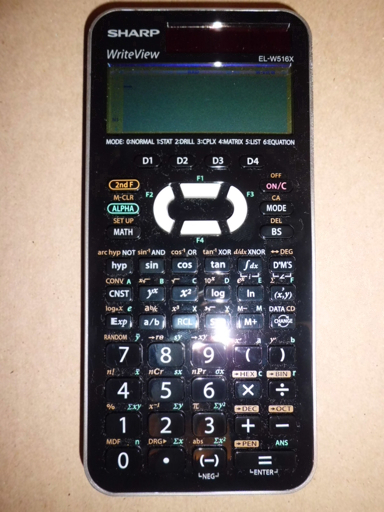1.Great review of the Sharp EL-W516x. I'm still trying to decide between the W516x and the Casio FX-115ES. I also have a new HP50g. I'm a former high school biology teacher soon going back to school to get a physics (or math) degree. I haven't had a need for a calculator in over 30 years:) I value your recommendations. I also have a HP 35s which seems really fun but all the bad reviews have made me reconsider.

2.How do you get rid of the annoying way that results are displayed? I mean, every result is given in fractional way. If you want to now five divided by six you get 5/6. It sucks!

1.I just figured out how to change mine so that it goes to decimal answers by default. Press MODE then 1 then 0 for "STAT 0 [SD]" mode. It displays answers in decimals and as far as I know, doesn't change anything else.

2.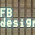No, it's not good. In STAT mode you have nor write view!
You can have write view only in normal mode, but then you only get fractional way of displaying division.
I would like WV with floating point display!
Everything else is good on thi calculator, EXCEPT THAT!
;(

3.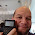Unfortunately, it is the default. To get the decimal answer, press CHANGE. I find this annoying too.

4.I punch in my equation and hit equals and then a +k appears after my equation and i get the wrong answer. What is up with that?

1.I am not sure Shane. I tried to duplicate the "+k" problem and could not. Can you please me what modes you were in and what equation you were trying to do that generated this problem?

Eddie

2.happens to me as well
it is really annoying

5.This comment has been removed by the author.

6.Eddie, I tried unsuccessfully to locate the LCM/GCD function on the Sharp EL-W516X. Does it even have this function? If so, How can it be accessed, would greatly appreciate any information on this.

1.James,

I am checking the catalog and do not see either the LCM or GCD function; they are not present in the Math menu. The manual doesn't mention these functions.

I would say that the EL-W516X does not have LCM or GCD.

Eddie

2.Nor does it have the FACTorial function or repeating decimal marker and a weak integrating function compaired to the casio fx-991es but it does have 4 formula memories, 4 definable keys, and a customizable shutdown screen and remembers everything on power up.

7.Nice blog it is informative thank you for sharing Tableau Online Training

### Calculator Python: Lambda Functions

Calculator Python: Lambda Functions Introduction to Lambda Functions Lambda functions are a quick, one expression, one line, python function...